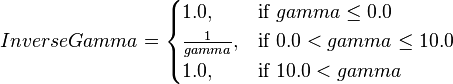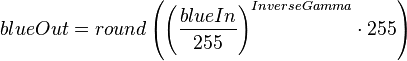## General

To adjust colors, brightness and contrast of a raster image or set a gamma value use the settings in the color dialog. It opens, when an image is selected.These settings are written to the file, but the image itself is not altered. Therefore you can use these filters not only for embedded images but for linked ones as well.

The colors are defined in a 8-bit RGB color space. The color of a pixel is a vector with a red component, a green component, and a blue component, each with integer values in the interval [0‥255].

A filter can be described by a function. Input parameter is the original color or the output of another filter. The resulting output is the rendered color or the input to another filter. You can illustrate the relationship between input color and output color by a function graph in a coordinate system with the input color on the x-axis and the output color on the y-axis. If no filter is applied, the function graph is a straight line in 45 degree to the x-axis.## Contrast

The contrast is a signed percent value in the interval [−100%‥100%]. A positive value increases the contrast of the rendered image, a negative value decreases the contrast. This filter is applied to all color channels at the same time.

The filter function is linear. Its slope is calculated by$slope = \begin{cases} \frac {128}{128-contrast \cdot 127}, & \mbox{if } contrast \ge 0 \\ \frac {128+contrast \cdot 127} {128}, & \mbox{if } contrast < 0 \end{cases}$

contrast adjustment positivecontrast adjustment negativeThe value 128 is not affected by this filter. So the resulting function has the term$output = slope \cdot (input - 128) + 128$

## Luminance

The luminance is a signed percent value in the interval [−100%‥100%]. A positive value increases the brightness of the rendered image, a negative value decreases the brightness. It is applied to all color channels at the same time. The function graph is simply shifted up or down.$output = input + luminance \cdot 255$.

The color adjustment is similar to the luminance, but has for each color channel a separate value. A positive value increases the intensity of the that color, a negative value decreases it. The color adjustment can be used for a white balance of the rendered image.

## Resulting Filter

Color adjustment, contrast and luminance settings are applied at the same time. Let blueIn be the blue component of a pixel in the original image, then the blue component of the rendered pixel can be calculated as below. The green and red components are calculated analogous.$raw = slope \cdot (blueIn-128)+128+bluePercent \cdot 255 + luminance \cdot 255$

The rendered color blueOut is rounded to integer and constrained to the interval [0‥255].$blueOut = \begin{cases} 0, & \mbox{if }raw <0 \\ round(raw), & \mbox{if }0 \le raw \le 255 \\ 255, & \mbox{if }255 < raw \end{cases}$

This filter is not applied to an alpha-channel, which might be present.

The adjustments here are applied before changes, which are induced by the gamma value.

## Gamma

The gamma filter provides a non-linear filter, which mainly affects the contrast of the mid-tones. It can be used for a manually gamma correction for special output devices or environments. This filter is applied to all color channels at the same time.

The value gamma is a floating point number. It is stored in the file as percent, e.g. the value 2.5 in the UI is stored as 250%. The UI constrains the value to 0.1 ≤ gamma ≤ 10. Internally values outside the interval ]0‥10] are forced to 1.$InverseGamma = \begin{cases} 1.0, & \mbox{if }gamma \le 0.0 \\ \frac {1}{gamma}, & \mbox{if }0.0

Let blueIn be the blue component of a pixel in the image after applied filter for colors, luminance and contrast as described above. Then the blue component of the rendered pixel can be calculated as below, the green and red components are calculated analogous.$blueOut = round \left ( \left ( \frac{blueIn}{255} \right)^{InverseGamma} \cdot 255 \right )$

Because 0InverseGamma=0 and 1InverseGamma=1, very dark colors and very bright colors are less affected than mid-tones.

This filter is not applied to an alpha-channel, if any.